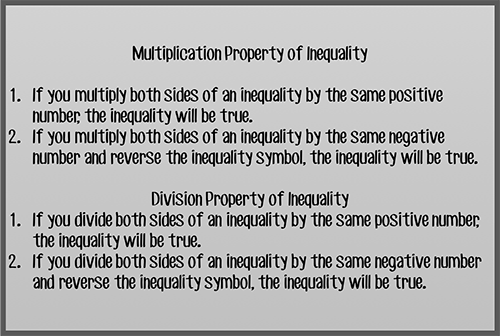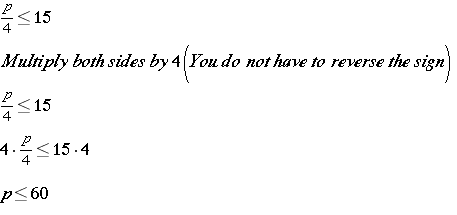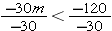# Solving Inequalities by Multiplying or Dividing

In this lesson, you will learn to solve inequalities that involve multiplying or dividing. Once again, you will use your knowledge of solving equations as a basis for solving inequalities. There is one important rule that will apply to inequality multiplication and division that involves negative numbers. Look carefully at the Properties Chart below to help you understand this important rule.Try a few of these to become familiar with this rule.

## Practice

#### Each time the sign is kept the same and the numbers multiplied. Check the inequality to see if the new inequality is accurate. It should be inaccurate any time the inequality is multiplied by a negative number.

Inequality: 3 < 4

Multiply each side by: 2

New Inequality: 6 < 8     accurate

Inequality: 5 > 2

Multiply each side by: -3

New Inequality: -15 > -6     inaccurate

Inequality: -1 ≤ 5

Multiply each side by: 4

New Inequality: -4 ≤ 20     accurate

Inequality: 3 ≥ -10

Multiply each side by: -2

New Inequality: -6 ≥ 20     inaccurate

Inequality: 1 < 8

Multiply each side by: -8

New Inequality: -8 < -64     inaccurate

Inequality: -3 ≤ 10

Multiply each side by: -2

New Inequality: 6 ≤ -20     inaccurate

Inequality: 4 < 8

Divide each side by: 4

New Inequality: 1 < 2     accurate

Inequality: 9 ≥ -12

Divide each side by: -3

New Inequality: -3 ≥ 4     inaccurate

Inequality: 20 > 10

Divide each side by: 5

New Inequality: 4 > 2     accurate

Inequality: -8 < -4

Divide each side by: -2

New Inequality: 4 < 2     inaccurate

Inequality: -24 ≤ 16

Divide each side by: -4

New Inequality: 6 ≤ -4     inaccurate

Inequality: 12 > -6

Divide each side by: -6

New Inequality: -2 > 1     inaccurateNow, we will solve an inequality by multiplying.

## Investigate

Four friends went out to lunch at a popular restaurant and decided to share the cost of the meal. Each person’s share is at most \$15. The inequalitycan be used to find p, the price in dollars of their lunch. What is the solution set of the inequality and what does it represent in this situation?

Strategy

Use inverse operations to solve the inequality just as you would solve an equation.Solution

Now, interpret the solution. This makes sense because if each person’s share was no more than \$15 each, then the entire lunch cannot cost more than \$60.

We should also take a look at an example of solving an inequality by dividing.

## InvestigateWebb is a scuba diver. His elevation compared to sea level changes at a constant rate of -30 feet per minute.  He started at the water’s surface, and his elevation is now less than -120 feet. How long has Webb been descending?

Strategy

For this one, you need to translate the words into an inequality. Let m represent the minutes that he has been descending.

Rate of change is (-30), multiplied by m (minutes) is less than (<) -120

-30m < -120

Use inverse operations to solve the inequality.Divide both sides by -30 AND reverse the inequality symbol.

m > 4

Solution

Interpret the solution set.  The question is asking how long he has been descending to have reached less than 120 feet below the surface, and m represents minutes.  This means he has been descending more than 4 minutes to have reached a level less than -120. Substitute a number from the solution set, 5 minutes.

-30(5) < -120

-150 < -120 Correct!

(source)